# 从生物神经元到人工神经元

ANN 的早期成功让人们广泛相信，人类马上就能造出真正的智能机器了。1960 年代，当这个想法落空时，资助神经网络的钱锐减，ANN 进入了寒冬。1980 年代早期，诞生了新的神经网络架构和新的训练方法，连结主义（研究神经网络）复苏，但是进展很慢。到了 1990 年代，出现了一批强大的机器学习方法，比如支持向量机（见第 05 章）。这些新方法的结果更优，也比 ANN 具有更扎实的理论基础，神经网络研究又一次进入寒冬。我们正在经历的是第三次神经网络浪潮。这波浪潮会像前两次那样吗？这次与前两次有所不同，这一次会对我们的生活产生更大的影响，理由如下：

• 我们现在有更多的数据，用于训练神经网络，在大而复杂的问题上，ANN 比其它 ML 技术表现更好；

• 自从 1990 年代，计算能力突飞猛进，现在已经可以在理想的时间内训练出大规模的神经网络了。一部分原因是摩尔定律（在过去 50 年间，集成电路中的组件数每两年就翻了一倍），另外要归功于游戏产业，后者生产出了强大的 GPU 显卡。还有，云平台使得任何人都能使用这些计算能力；

• 训练算法得到了提升。虽然相比 1990 年代，算法变化不大，但这一点改进却产生了非常大的影响；

• 在实践中，人工神经网络的一些理论局限没有那么强。例如，许多人认为人工神经网络训练算法效果一般，因为它们很可能陷入局部最优，但事实证明，这在实践中是相当罕见的（或者如果它发生，它们也通常相当接近全局最优）；

• ANN 已经进入了资助和进步的良性循环。基于 ANN 的惊艳产品常常上头条，从而吸引了越来越多的关注和资金，促进越来越多的进步和更惊艳的产品。

## 生物神经元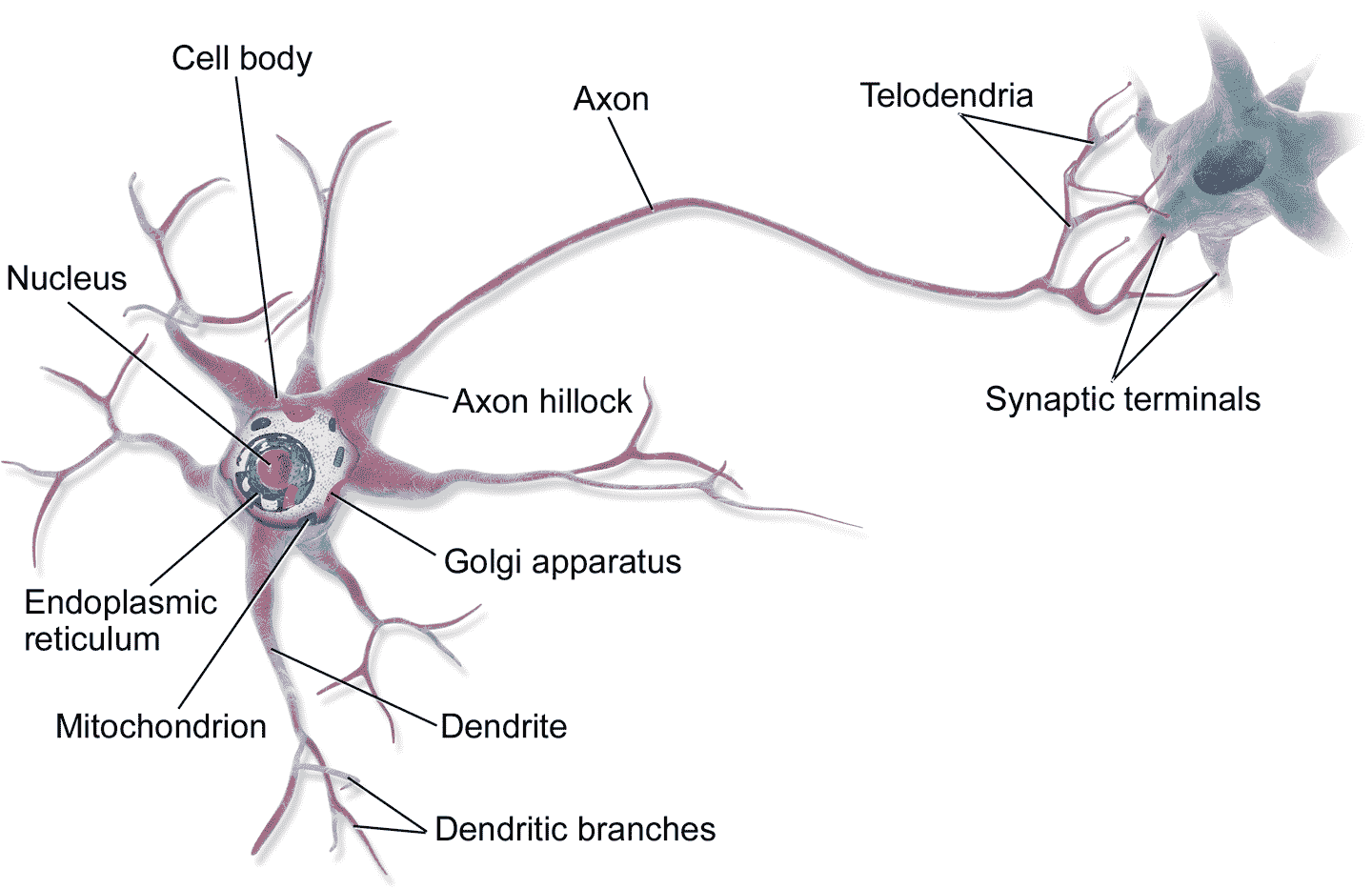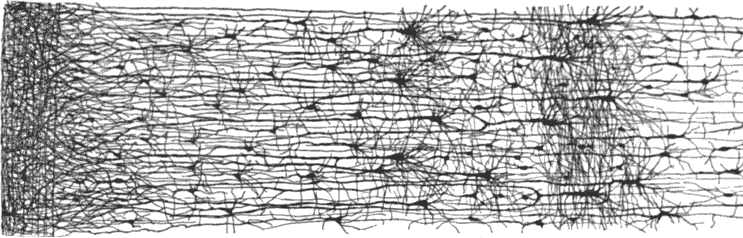## 神经元的逻辑计算

McCulloch 和 Pitts 提出了一个非常简单的生物神经元模型，它后来演化成了人工神经元：一个或多个二元（开或关）输入，一个二元输出。当达到一定的输入量时，神经元就会产生输出。在论文中，两位作者证明就算用如此简单的模型，就可以搭建一个可以完成任何逻辑命题计算的神经网络。为了展示网络是如何运行的，我们自己亲手搭建一些不同逻辑计算的 ANN（见图 10-3），假设有两个活跃的输入时，神经元就被激活。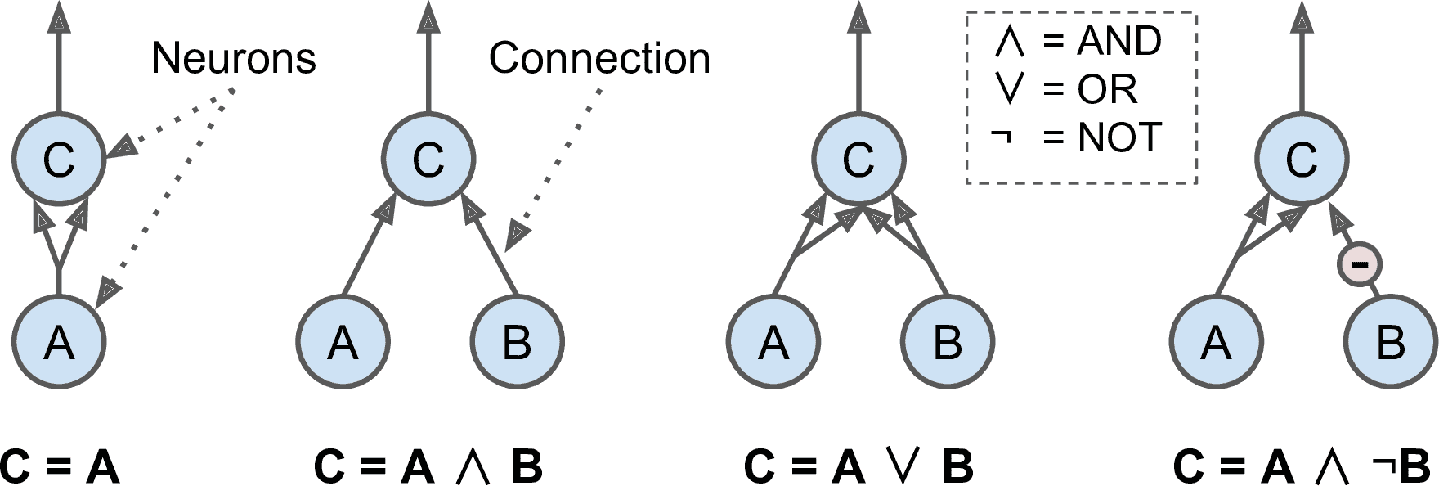• 左边第一个网络是确认函数：如果神经元A被激活，那么神经元C也被激活（因为它接收来自神经元A的两个输入信号），但是如果神经元A关闭，那么神经元C也关闭。

• 第二个网络执行逻辑 AND：神经元C只有在激活神经元AB（单个输入信号不足以激活神经元C）时才被激活。

• 第三个网络执行逻辑 OR：如果神经元A或神经元B被激活（或两者），神经元C被激活。

• 最后，如果我们假设输入连接可以抑制神经元的活动（生物神经元是这样的情况），那么第四个网络计算一个稍微复杂的逻辑命题：如果神经元B关闭，只有当神经元A是激活的，神经元C才被激活。如果神经元A始终是激活的，那么你得到一个逻辑 NOT：神经元C在神经元B关闭时是激活的，反之亦然。

## 感知机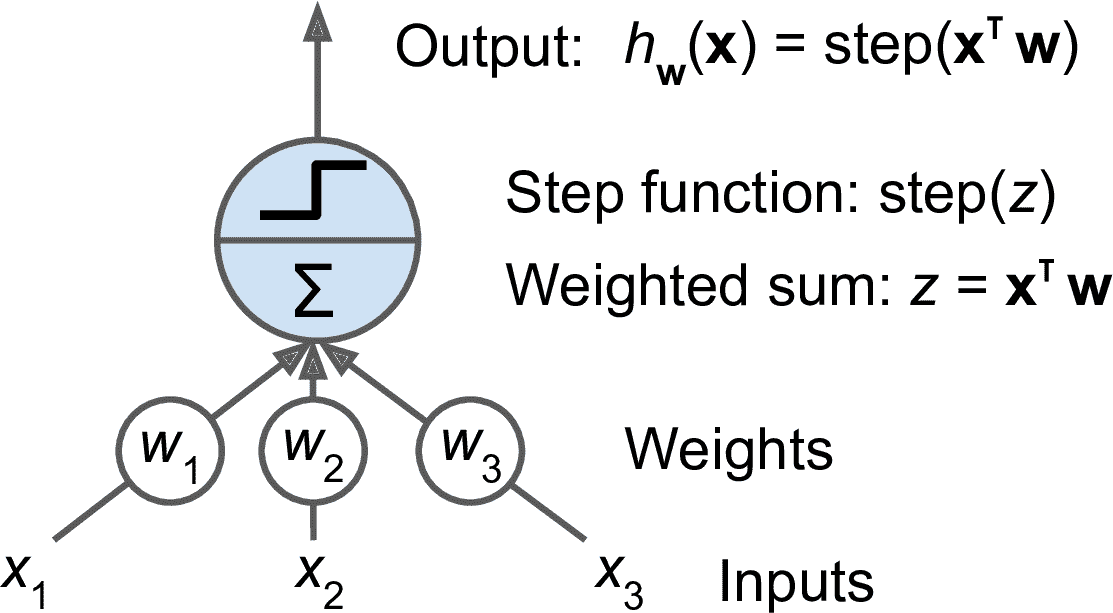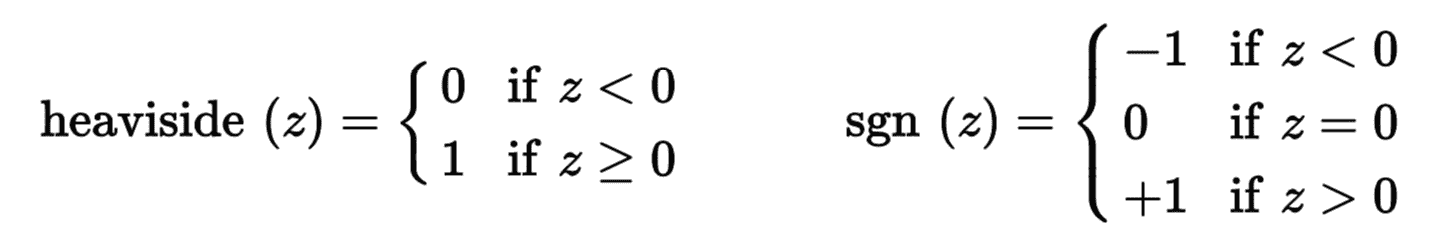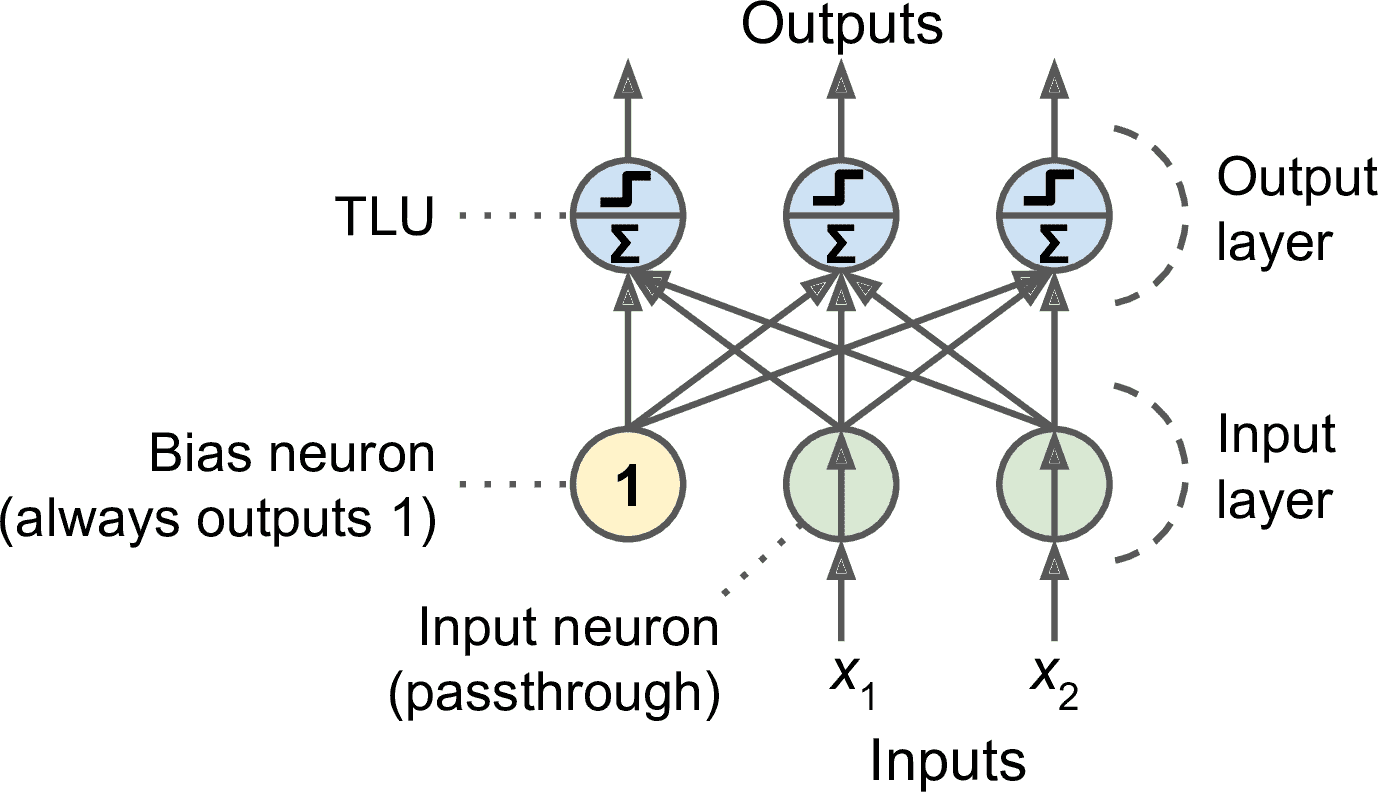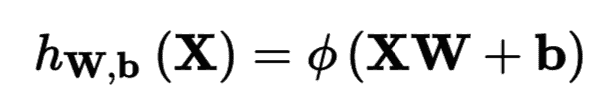• X表示输入特征矩阵，每行是一个实例，每列是一个特征；

• 权重矩阵W包含所有的连接权重，除了偏置神经元。每有一个输入神经元权重矩阵就有一行，神经层每有一个神经元权重矩阵就有一列；

• 偏置向量b含有所有偏置神经元和人工神经元的连接权重。每有一个人工神经元就对应一个偏置项；

• 函数φ被称为激活函数，当人工神经网络是 TLU 时，激活函数是阶跃函数（后面会讨论更多的激活函数）。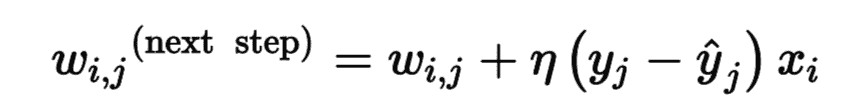• 其中w[i, j]是第i个输入神经元与第j个输出神经元之间的连接权重；

• x[i]是当前训练实例的第i个输入值；

• y_hat[j]是当前训练实例的第j个输出神经元的输出；

• y[j]是当前训练实例的第j个输出神经元的目标输出；

• η是学习率。

Scikit-Learn 提供了一个Perceptron类，它实现了一个 单 TLU 网络。它可以实现大部分功能，例如用于鸢尾花数据集（第 4 章中介绍过）：

import numpy as np
from sklearn.linear_model import Perceptron

X = iris.data[:, (2, 3)]  # petal length, petal width
y = (iris.target == 0).astype(np.int)  # Iris setosa?

per_clf = Perceptron()
per_clf.fit(X, y)

y_pred = per_clf.predict([[2, 0.5]])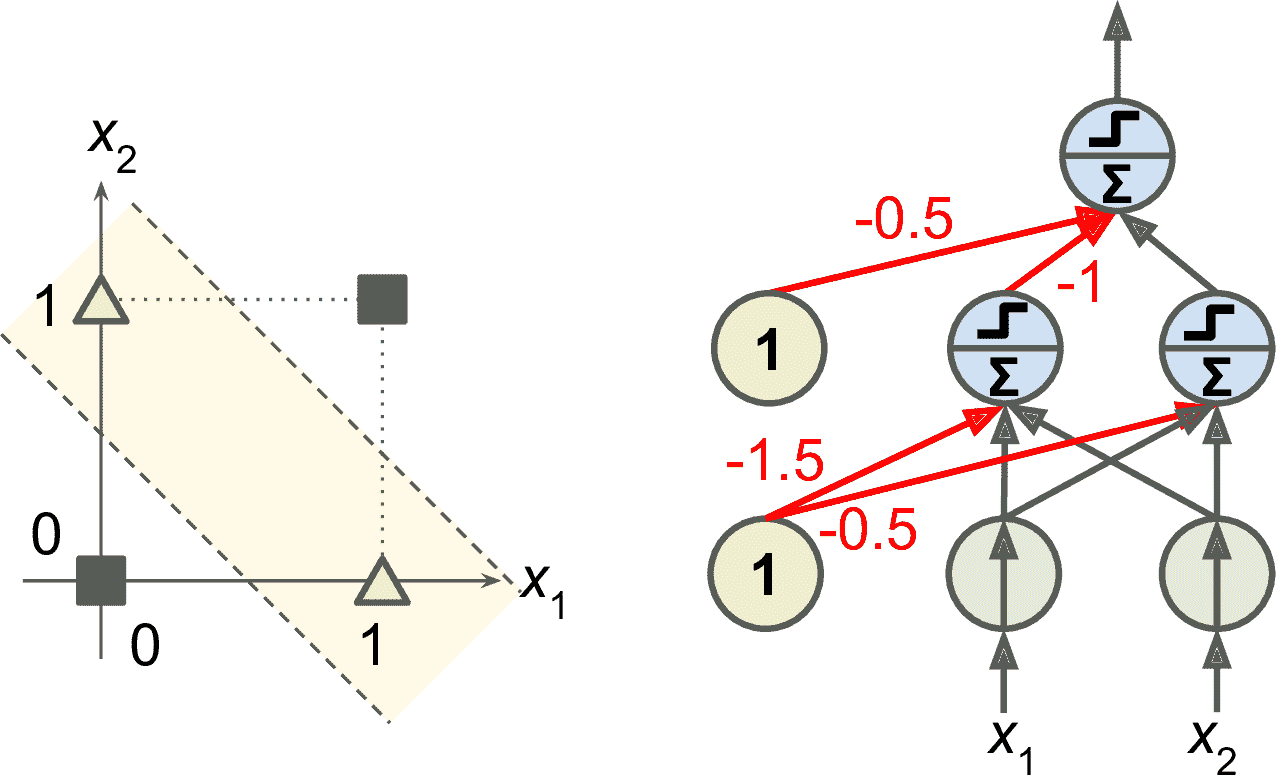## 多层感知机与反向传播

MLP 由一个输入层、一个或多个称为隐藏层的 TLU 组成，一个 TLU 层称为输出层（见图 10-7）。靠近输入层的层，通常被称为浅层，靠近输出层的层通常被称为上层。除了输出层，每一层都有一个偏置神经元，并且全连接到下一层。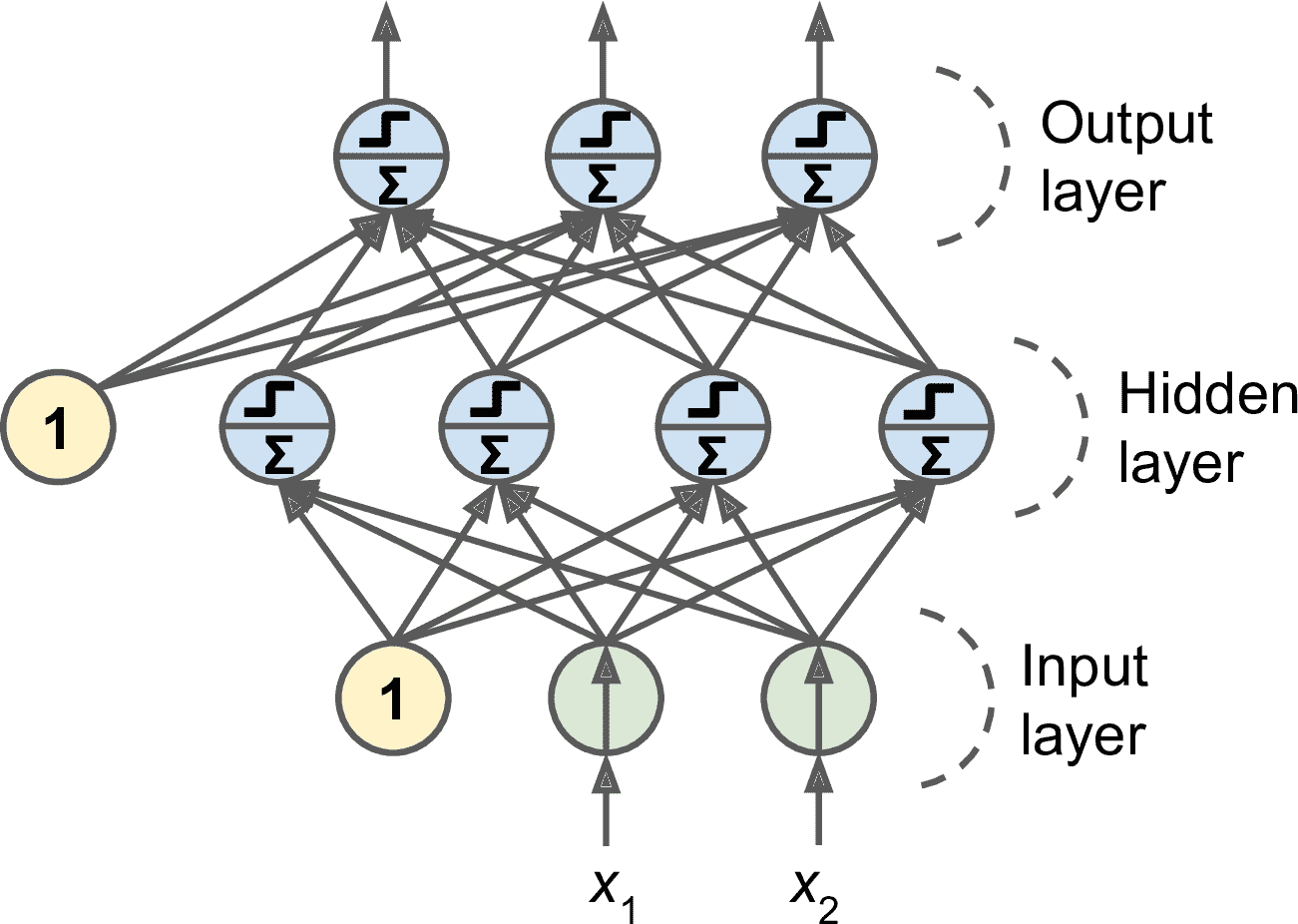• 每次处理一个微批次（假如每个批次包含 32 个实例），用训练集多次训练 BP，每次被称为一个周期（epoch）；

• 每个微批次先进入输入层，输入层再将其发到第一个隐藏层。计算得到该层所有神经元的（微批次的每个实例的）输出。输出接着传到下一层，直到得到输出层的输出。这个过程就是前向传播：就像做预测一样，只是保存了每个中间结果，中间结果要用于反向传播；

• 然后计算输出误差（使用损失函数比较目标值和实际输出值，然后返回误差）；

• 接着，计算每个输出连接对误差的贡献量。这是通过链式法则（就是对多个变量做微分的方法）实现的；

• 然后还是使用链式法则，计算最后一个隐藏层的每个连接对误差的贡献，这个过程不断向后传播，直到到达输入层。

• 最后，BP 算法做一次梯度下降步骤，用刚刚计算的误差梯度调整所有连接权重。

BP 算法十分重要，再归纳一下：对每个训练实例，BP 算法先做一次预测（前向传播），然后计算误差，然后反向通过每一层以测量误差贡献量（反向传播），最后调整所有连接权重以降低误差（梯度下降）。（译者注：我也总结下吧，每次训练都先是要设置周期数，每个周期其实做的就是三件事，向前传一次，向后传一次，然后调整参数，接着再进行下一周期。）

• 双曲正切函数： tanh (z) = 2σ(2z) – 1

• ReLU 函数：ReLU(z) = max(0, z)

ReLU 函数是连续的，但是在z=0时不可微（斜率突然改变，导致梯度下降在 0 点左右跳跃），ReLU 的变体是当z<0时，z=0。但在实践中，ReLU 效果很好，并且具有计算快速的优点，于是成为了默认激活函数。最重要的是，它没有最大输出值，这有助于减少梯度下降期间的一些问题（第 11 章再介绍）。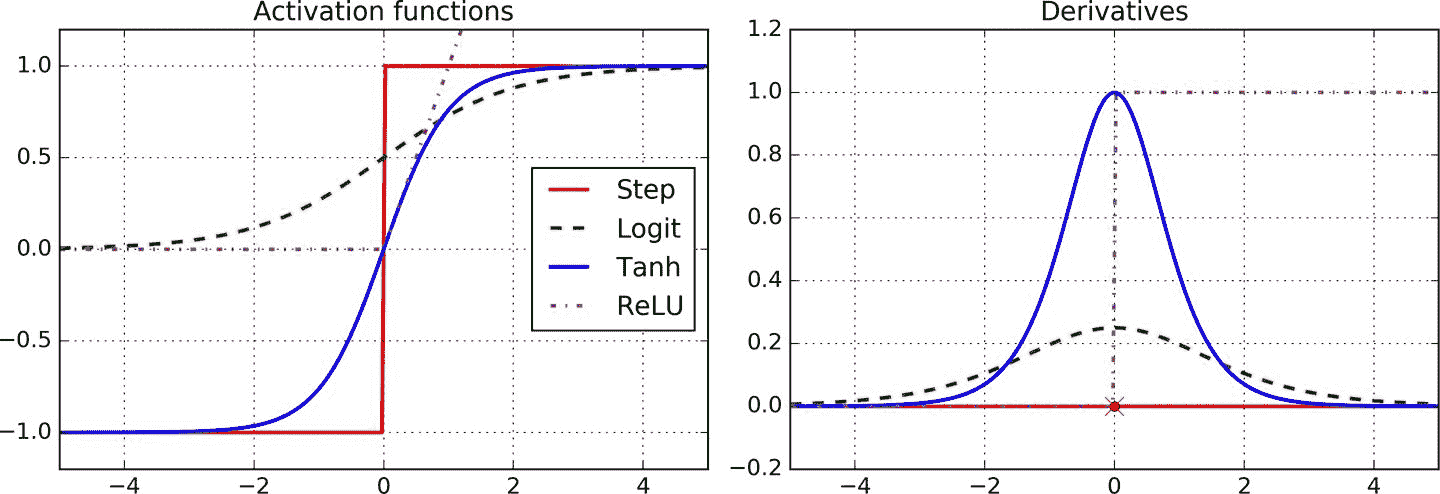## 回归 MLP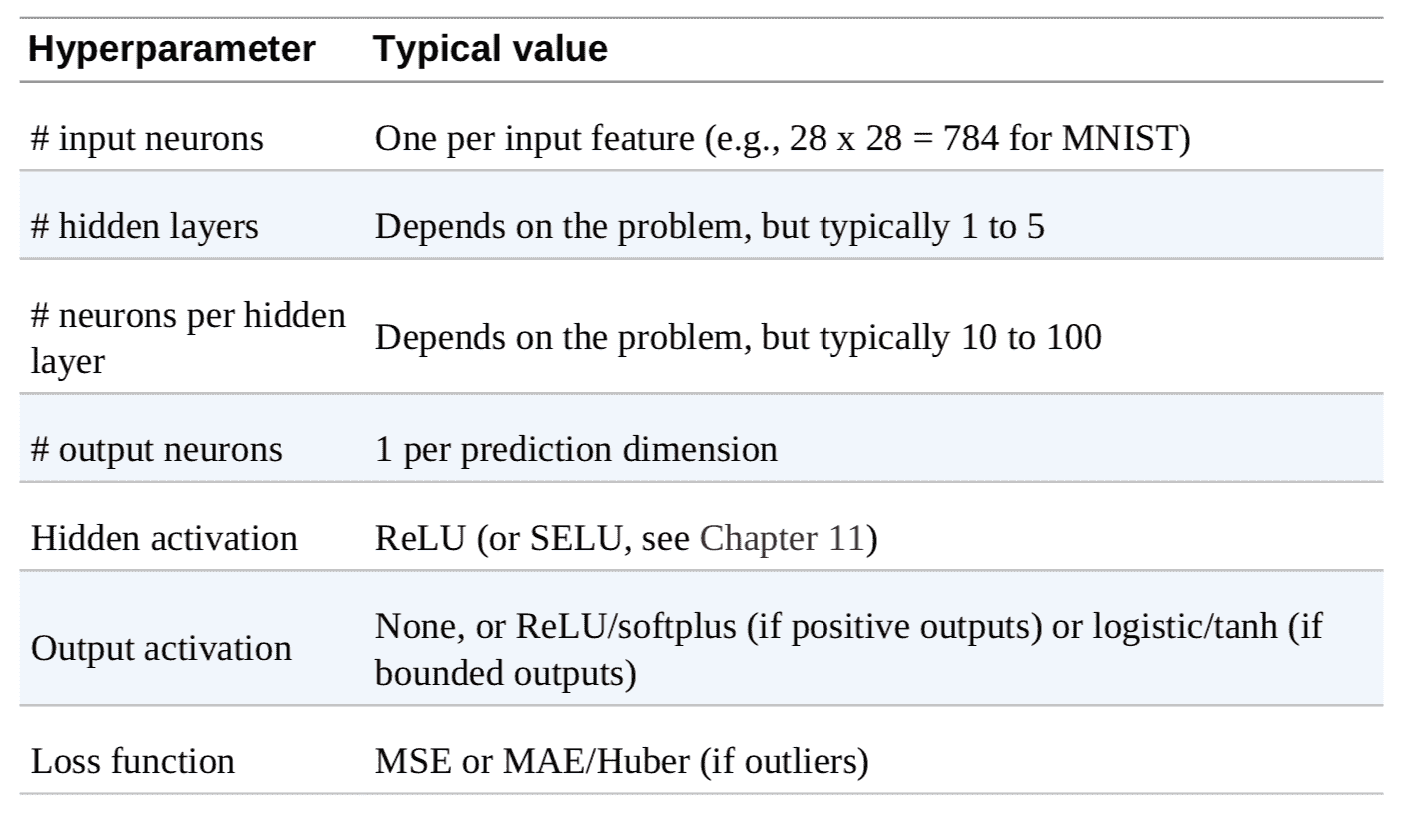## 分类 MLP

MLP 也可用于分类，对于二元分类问题，只需要一个使用 Logistic 激活的输出神经元：输出是一个 0 和 1 之间的值，作为正类的估计概率。

MLP 也可以处理多标签二元分类（见第 3 章）。例如，邮件分类系统可以预测一封邮件是垃圾邮件，还是正常邮件，同时预测是紧急，还是非紧急邮件。这时，就需要两个输出神经元，两个都是用 Logistic 函数：第一个输出垃圾邮件的概率，第二个输出紧急的概率。更为一般的讲，需要为每个正类配一个输出神经元。多个输出概率的和不一定非要等于 1。这样模型就可以输出各种标签的组合：非紧急非垃圾邮件、紧急非垃圾邮件、非紧急垃圾邮件、紧急垃圾邮件。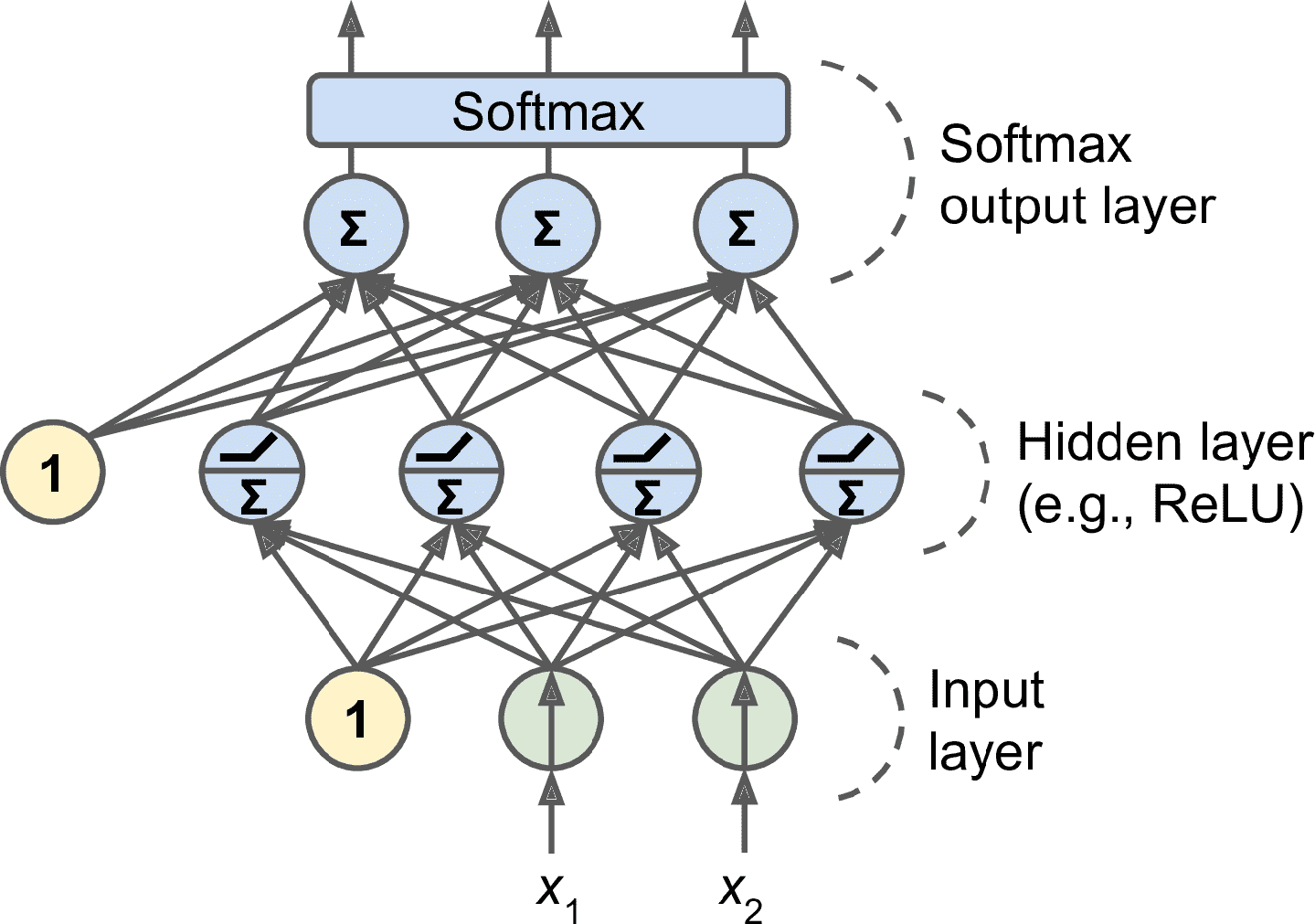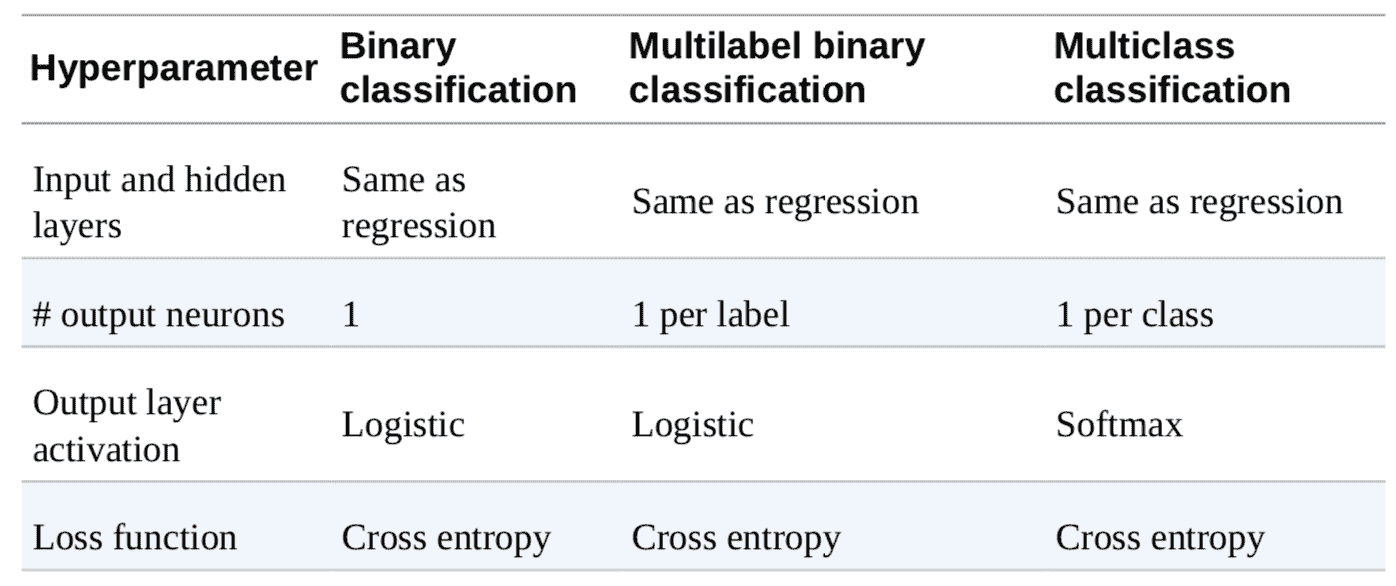# 用 Keras 实现 MLP

Keras 是一个深度学习高级 API，可以用它轻松地搭建、训练、评估和运行各种神经网络。Keras 的文档见这里Keras 参考实现是 François Chollet 开发的，于 2015 年 3 月开源。得益于 Keras 简单易用灵活优美，迅速流行开来。为了进行神经网络计算，必须要有计算后端的支持。目前可选三个流行库：TensorFlow、CNTK 和 Theano。为避免误会，将 GitHub 上的 Keras 参考实现称为多后端 Keras。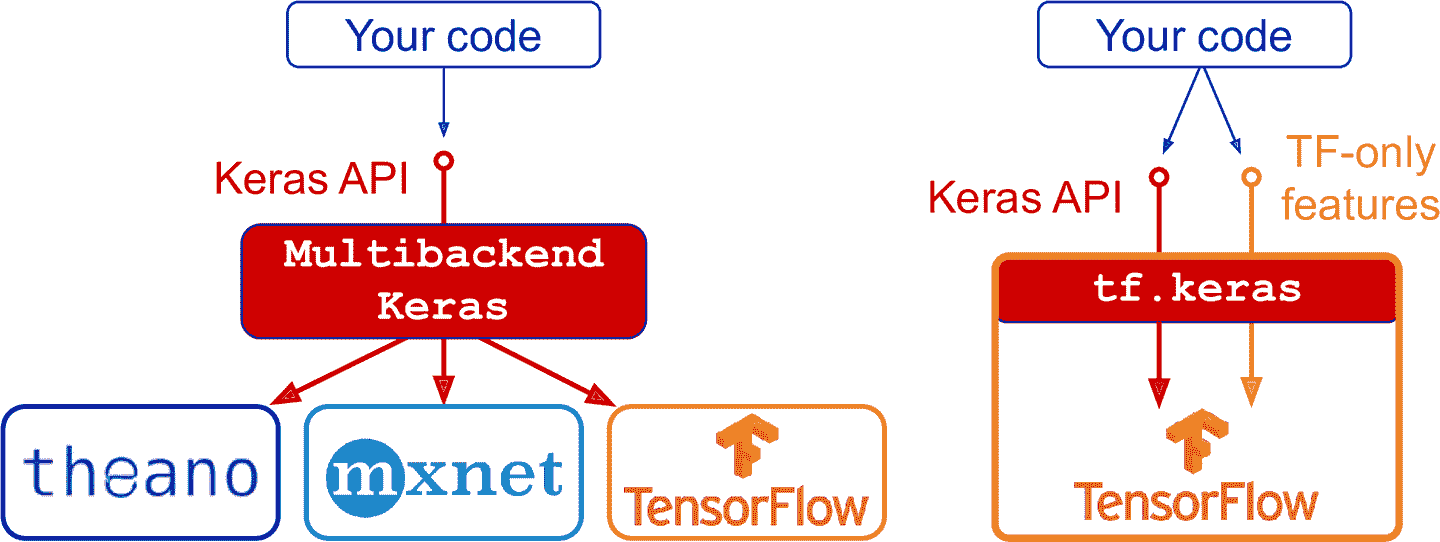## 安装 TensorFlow 2

$cd$ML_PATH                 # Your ML working directory (e.g., $HOME/ml)$ source my_env/bin/activate  # on Linux or macOS
$.\my_env\Scripts\activate # on Windows  然后安装 TensorFlow 2（如果没有使用虚拟环境，需要管理员权限，或加上选项--user）: $ python3 -m pip install --upgrade tensorflow


>>> import tensorflow as tf
>>> from tensorflow import keras
>>> tf.__version__
'2.0.0'
>>> keras.__version__
'2.2.4-tf'


## 使用顺序 API 创建图片分类器

### 使用 Keras 加载数据集

Keras 提供一些实用的函数用来获取和加载常见的数据集，包括 MNIST、Fashion MNIST 和第 2 章用过的加州房产数据集。加载 Fashion MNIST：

fashion_mnist = keras.datasets.fashion_mnist
(X_train_full, y_train_full), (X_test, y_test) = fashion_mnist.load_data()


>>> X_train_full.shape
(60000, 28, 28)
>>> X_train_full.dtype
dtype('uint8')


X_valid, X_train = X_train_full[:5000] / 255.0, X_train_full[5000:] / 255.0
y_valid, y_train = y_train_full[:5000], y_train_full[5000:]


class_names = ["T-shirt/top", "Trouser", "Pullover", "Dress", "Coat",
"Sandal", "Shirt", "Sneaker", "Bag", "Ankle boot"]


>>> class_names[y_train]
'Coat'### 用顺序 API 创建模型

model = keras.models.Sequential()


• 第一行代码创建了一个顺序 模型，这是 Keras 最简单的模型，是由单层神经元顺序连起来的，被称为顺序 API；

• 接下来创建了第一层，这是一个Flatten层，它的作用是将每个输入图片转变为 1D 数组：如果输入数据是X，该层则计算X.reshape(-1, 1)。该层没有任何参数，只是做一些简单预处理。因为是模型的第一层，必须要指明input_shapeinput_shape不包括批次大小，只是实例的形状。另外，第一层也可以是keras.layers.InputLayer，设置input_shape=[28,28];

• 然后，添加了一个有 300 个神经元的紧密层，激活函数是 ReLU。每个紧密层只负责自身的权重矩阵，权重矩阵是神经元与输入的所有连接权重。紧密层还要负责偏置项（每个神经元都有一个偏置项）向量。当紧密层收到输入数据时，就利用公式 10-2 进行计算；

• 接着再添加第二个紧密层，激活函数仍然是 ReLU；

• 最后，加上一个拥有 10 个神经元的输出层（每有一个类就要有一个神经元），激活函数是 softmax（保证输出的概率和等于 1，因为就只有这是个类，具有排他性）。

model = keras.models.Sequential([
keras.layers.Flatten(input_shape=[28, 28]),
keras.layers.Dense(300, activation="relu"),
keras.layers.Dense(100, activation="relu"),
keras.layers.Dense(10, activation="softmax")
])


#### 使用 KERAS.IO 的代码实例

keras.io 上的代码也可以用于tf.keras，但是需要修改引入。例如，对于下面的代码：

py from keras.layers import Dense output_layer = Dense(10)

py from tensorflow.keras.layers import Dense output_layer = Dense(10)

py from tensorflow import keras output_layer = keras.layers.Dense(10)

>>> model.summary()
Model: "sequential"
_________________________________________________________________
Layer (type)                 Output Shape              Param #
=================================================================
flatten (Flatten)            (None, 784)               0
_________________________________________________________________
dense (Dense)                (None, 300)               235500
_________________________________________________________________
dense_1 (Dense)              (None, 100)               30100
_________________________________________________________________
dense_2 (Dense)              (None, 10)                1010
=================================================================
Total params: 266,610
Trainable params: 266,610
Non-trainable params: 0
_________________________________________________________________


>>> model.layers
[<tensorflow.python.keras.layers.core.Flatten at 0x132414e48>,
<tensorflow.python.keras.layers.core.Dense at 0x1324149b0>,
<tensorflow.python.keras.layers.core.Dense at 0x1356ba8d0>,
<tensorflow.python.keras.layers.core.Dense at 0x13240d240>]
>>> hidden1 = model.layers
>>> hidden1.name
'dense'
>>> model.get_layer('dense') is hidden1
True


>>> weights, biases = hidden1.get_weights()
>>> weights
array([[ 0.02448617, -0.00877795, -0.02189048, ..., -0.02766046,
0.03859074, -0.06889391],
...,
[-0.06022581,  0.01577859, -0.02585464, ..., -0.00527829,
0.00272203, -0.06793761]], dtype=float32)
>>> weights.shape
(784, 300)
>>> biases
array([0., 0., 0., 0., 0., 0., 0., 0., 0., ...,  0., 0., 0.], dtype=float32)
>>> biases.shape
(300,)


### 编译模型

model.compile(loss="sparse_categorical_crossentropy",
optimizer="sgd",
metrics=["accuracy"])


### 训练和评估模型

>>> history = model.fit(X_train, y_train, epochs=30,
...                     validation_data=(X_valid, y_valid))
...
Train on 55000 samples, validate on 5000 samples
Epoch 1/30
55000/55000 [======] - 3s 49us/sample - loss: 0.7218     - accuracy: 0.7660
- val_loss: 0.4973 - val_accuracy: 0.8366
Epoch 2/30
55000/55000 [======] - 2s 45us/sample - loss: 0.4840     - accuracy: 0.8327
- val_loss: 0.4456 - val_accuracy: 0.8480
[...]
Epoch 30/30
55000/55000 [======] - 3s 53us/sample - loss: 0.2252     - accuracy: 0.9192
- val_loss: 0.2999 - val_accuracy: 0.8926


fit()方法会返回History对象，包含：训练参数（history.params）、周期列表（history.epoch）、以及最重要的包含训练集和验证集的每个周期后的损失和指标的字典（history.history）。如果用这个字典创建一个 pandas 的DataFrame，然后使用方法plot()，就可以画出学习曲线，见图 10-12：

import pandas as pd
import matplotlib.pyplot as plt

pd.DataFrame(history.history).plot(figsize=(8, 5))
plt.grid(True)
plt.gca().set_ylim(0, 1) # set the vertical range to [0-1]
plt.show()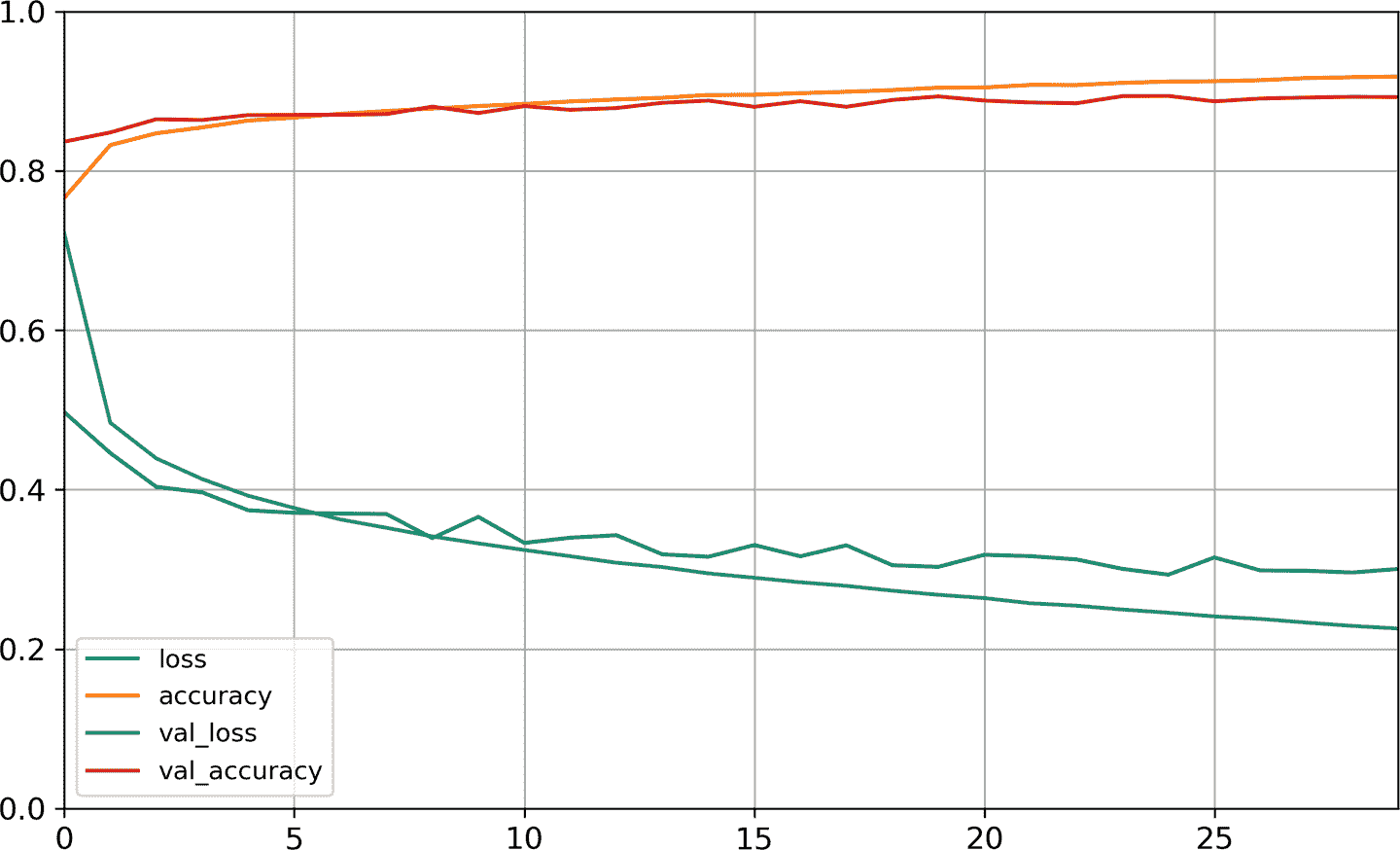>>> model.evaluate(X_test, y_test)
10000/10000 [==========] - 0s 29us/sample - loss: 0.3340 - accuracy: 0.8851
[0.3339798209667206, 0.8851]


### 使用模型进行预测

>>> X_new = X_test[:3]
>>> y_proba = model.predict(X_new)
>>> y_proba.round(2)
array([[0\.  , 0\.  , 0\.  , 0\.  , 0\.  , 0.03, 0\.  , 0.01, 0\.  , 0.96],
[0\.  , 0\.  , 0.98, 0\.  , 0.02, 0\.  , 0\.  , 0\.  , 0\.  , 0\.  ],
[0\.  , 1\.  , 0\.  , 0\.  , 0\.  , 0\.  , 0\.  , 0\.  , 0\.  , 0\.  ]],
dtype=float32)


>>> y_pred = model.predict_classes(X_new)
>>> y_pred
array([9, 2, 1])
>>> np.array(class_names)[y_pred]
array(['Ankle boot', 'Pullover', 'Trouser'], dtype='<U11')


>>> y_new = y_test[:3]
>>> y_new
array([9, 2, 1])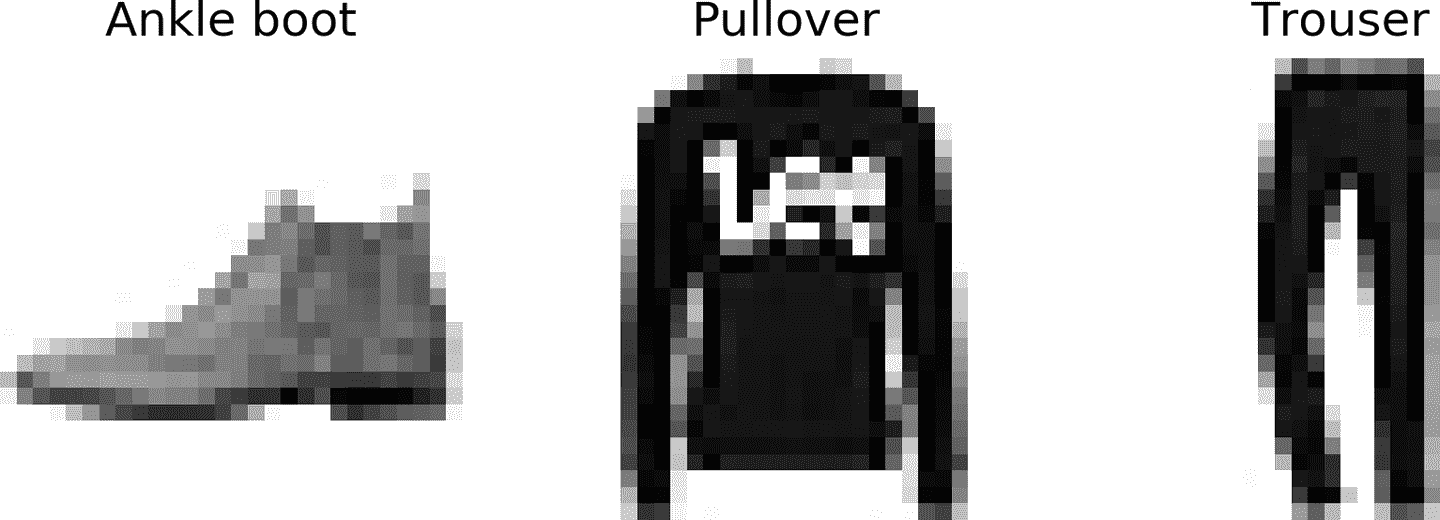## 使用顺序 API 搭建回归 MLP

from sklearn.datasets import fetch_california_housing
from sklearn.model_selection import train_test_split
from sklearn.preprocessing import StandardScaler

housing = fetch_california_housing()

X_train_full, X_test, y_train_full, y_test = train_test_split(
housing.data, housing.target)
X_train, X_valid, y_train, y_valid = train_test_split(
X_train_full, y_train_full)

scaler = StandardScaler()
X_train = scaler.fit_transform(X_train)
X_valid = scaler.transform(X_valid)
X_test = scaler.transform(X_test)


model = keras.models.Sequential([
keras.layers.Dense(30, activation="relu", input_shape=X_train.shape[1:]),
keras.layers.Dense(1)
])
model.compile(loss="mean_squared_error", optimizer="sgd")
history = model.fit(X_train, y_train, epochs=20,
validation_data=(X_valid, y_valid))
mse_test = model.evaluate(X_test, y_test)
X_new = X_test[:3] # pretend these are new instances
y_pred = model.predict(X_new)


## 使用函数式 API 搭建复杂模型

Wide & Deep 是一个非序列化的神经网络模型。这个架构是 Heng-Tze Cheng 在 2016 年在论文中提出来的。这个模型可以将全部或部分输入与输出层连起来，见图 10-14。这样，就可以既学到深层模式（使用深度路径）和简单规则（使用短路径）。作为对比，常规 MLP 会强制所有数据流经所有层，因此数据中的简单模式在多次变换后会被扭曲。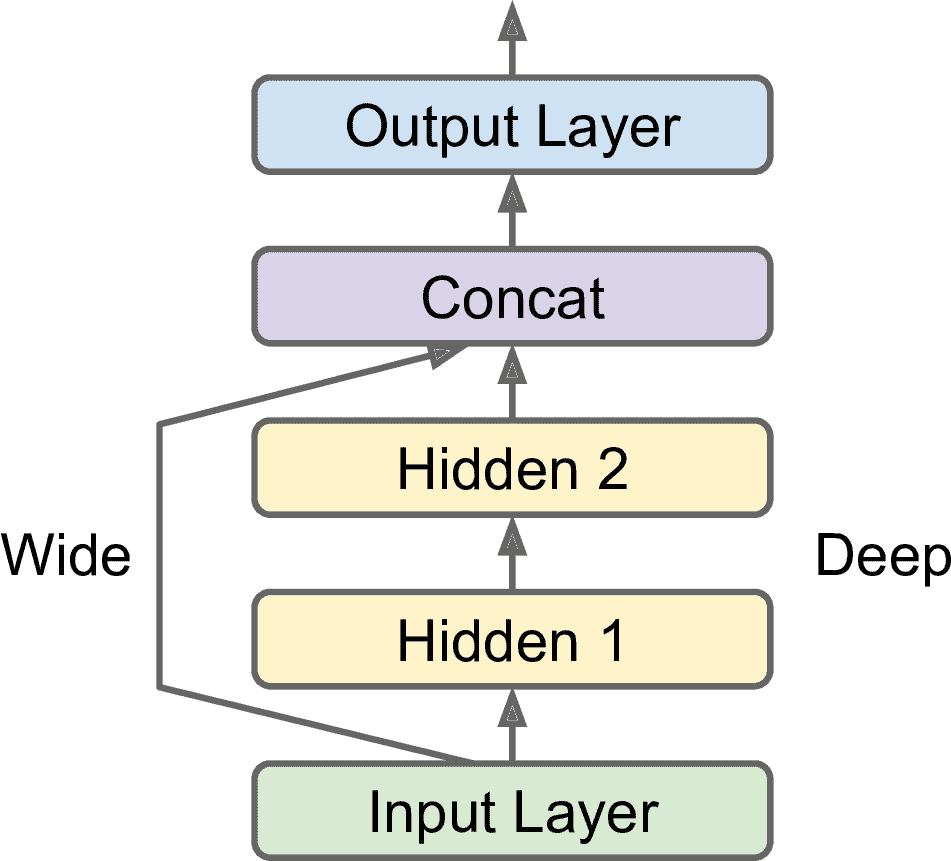input_ = keras.layers.Input(shape=X_train.shape[1:])
hidden1 = keras.layers.Dense(30, activation="relu")(input_)
hidden2 = keras.layers.Dense(30, activation="relu")(hidden1)
concat = keras.layers.Concatenate()([input_, hidden2])
output = keras.layers.Dense(1)(concat)
model = keras.Model(inputs=[input_], outputs=[output])


• 首先创建一个Input对象。包括模型输入的形状shape和数据类型dtype。模型可能会有多种输入。

• 然后，创建一个有 30 个神经元的紧密层，激活函数是 ReLU。创建好之后，将其作为函数，直接将输入传给它。这就是函数式 API 的得名原因。这里只是告诉 Keras 如何将层连起来，并没有导入实际数据。

• 然后创建第二个隐藏层，还是将其作为函数使用，输入时第一个隐藏层的输出；

• 接着，创建一个连接Concatenate层，也是作为函数使用，将输入和第二个隐藏层的输出连起来。可以使用keras.layers.concatenate()

• 然后创建输出层，只有一个神经元，没有激活函数，将连接层的输出作为输入。

• 最后，创建一个 Keras 的Model，指明输入和输出。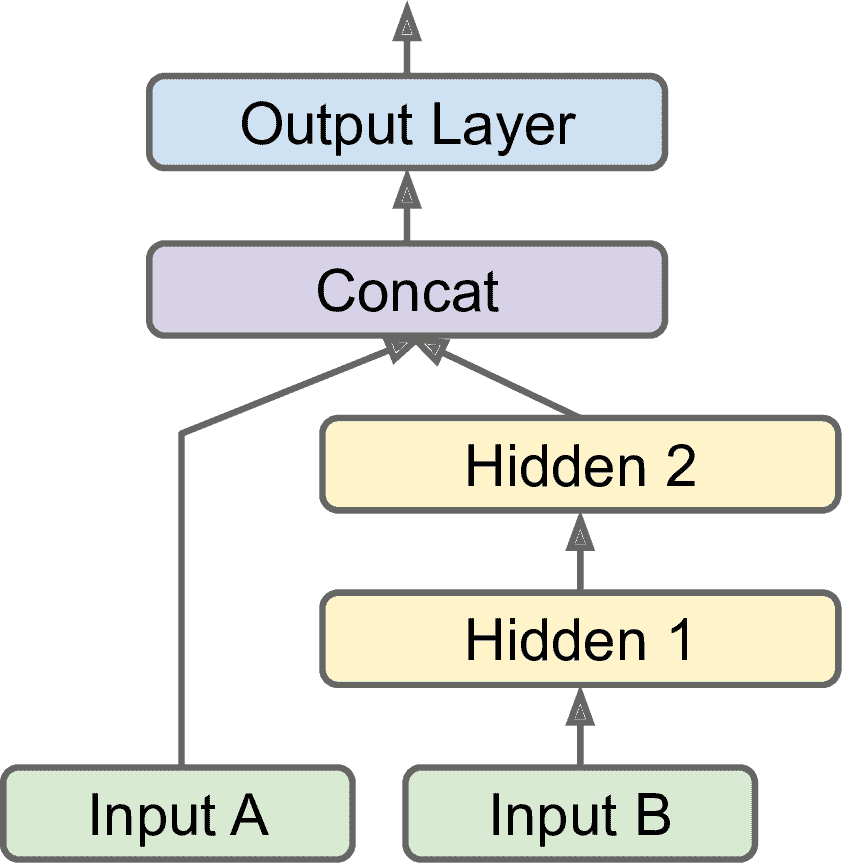input_A = keras.layers.Input(shape=, name="wide_input")
input_B = keras.layers.Input(shape=, name="deep_input")
hidden1 = keras.layers.Dense(30, activation="relu")(input_B)
hidden2 = keras.layers.Dense(30, activation="relu")(hidden1)
concat = keras.layers.concatenate([input_A, hidden2])
output = keras.layers.Dense(1, name="output")(concat)
model = keras.Model(inputs=[input_A, input_B], outputs=[output])


model.compile(loss="mse", optimizer=keras.optimizers.SGD(lr=1e-3))

X_train_A, X_train_B = X_train[:, :5], X_train[:, 2:]
X_valid_A, X_valid_B = X_valid[:, :5], X_valid[:, 2:]
X_test_A, X_test_B = X_test[:, :5], X_test[:, 2:]
X_new_A, X_new_B = X_test_A[:3], X_test_B[:3]

history = model.fit((X_train_A, X_train_B), y_train, epochs=20,
validation_data=((X_valid_A, X_valid_B), y_valid))
mse_test = model.evaluate((X_test_A, X_test_B), y_test)
y_pred = model.predict((X_new_A, X_new_B))


• 任务要求。例如，你想定位和分类图片中的主要物体。这既是一个回归任务（找到目标中心的坐标、宽度和高度）和分类任务。

• 相似的，对于相同的数据，你可能有多个独立的任务。当然可以每个任务训练一个神经网络，但在多数情况下，同时对所有任务训练一个神经网络，每个任务一个输出，后者的效果更好。这是因为神经网络可以在不同任务间学习有用的数据特征。例如，在人脸的多任务分类时，你可以用一个输出做人物表情的分类（微笑惊讶等等），用另一个输出判断是否戴着眼镜。

• 另一种情况是作为一种正则的方法（即，一种降低过拟合和提高泛化能力的训练约束）。例如，你想在神经网络中加入一些辅助输出（见图 10-16），好让神经网络的一部分依靠自身就能学到一些东西。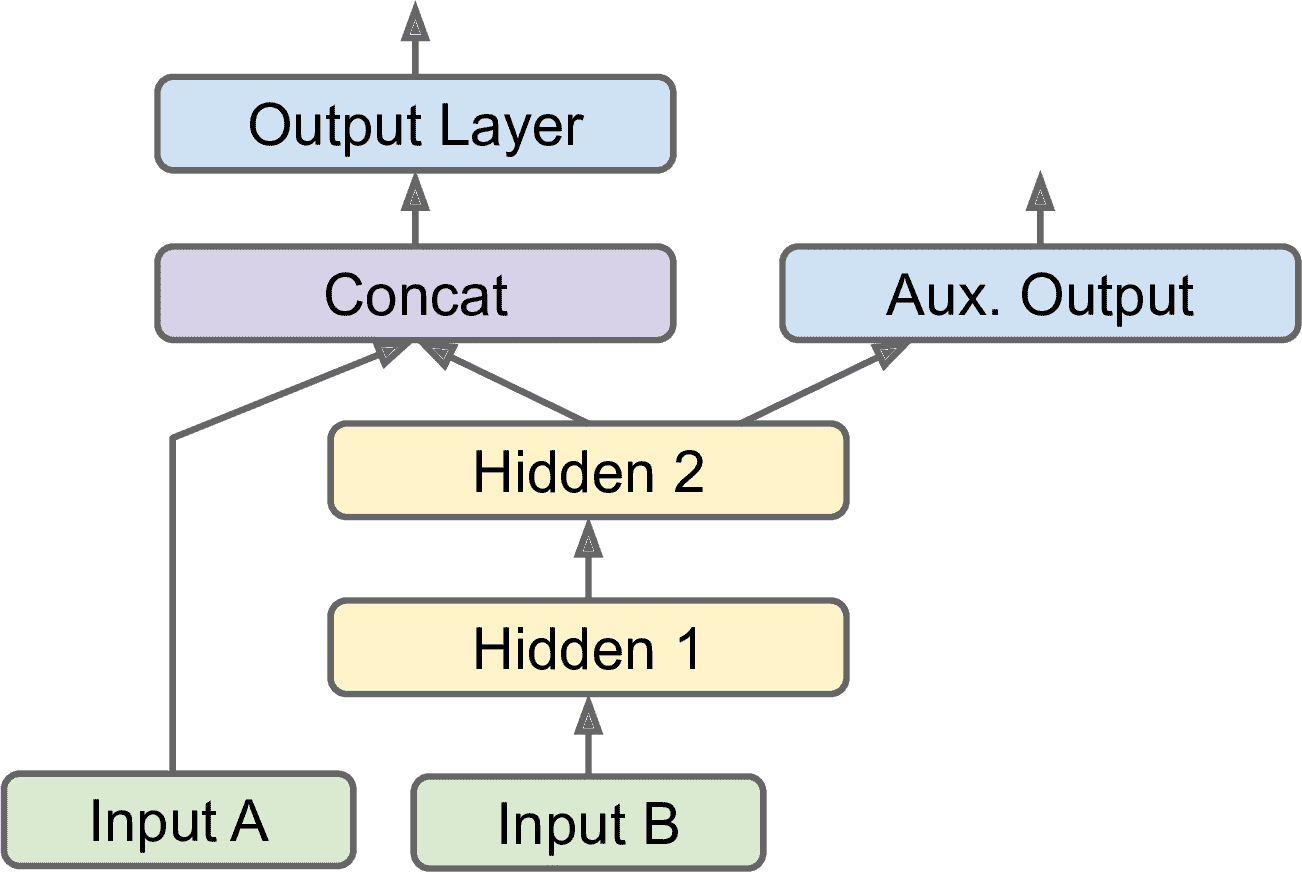[...] # output 层前面都一样
output = keras.layers.Dense(1, name="main_output")(concat)
aux_output = keras.layers.Dense(1, name="aux_output")(hidden2)
model = keras.Model(inputs=[input_A, input_B], outputs=[output, aux_output])


model.compile(loss=["mse", "mse"], loss_weights=[0.9, 0.1], optimizer="sgd")


history = model.fit(
[X_train_A, X_train_B], [y_train, y_train], epochs=20,
validation_data=([X_valid_A, X_valid_B], [y_valid, y_valid]))


total_loss, main_loss, aux_loss = model.evaluate(
[X_test_A, X_test_B], [y_test, y_test])


y_pred_main, y_pred_aux = model.predict([X_new_A, X_new_B])


## 使用子类化 API 搭建动态模型

Model类划分子类，在构造器中创建需要的层，调用call()进行计算。例如，创建一个下面的WideAndDeepModel类的实例，就可以创建与前面函数式 API 例子的同样模型，同样可以进行编译、评估、预测：

class WideAndDeepModel(keras.Model):
def __init__(self, units=30, activation="relu", **kwargs):
super().__init__(**kwargs) # handles standard args (e.g., name)
self.hidden1 = keras.layers.Dense(units, activation=activation)
self.hidden2 = keras.layers.Dense(units, activation=activation)
self.main_output = keras.layers.Dense(1)
self.aux_output = keras.layers.Dense(1)

def call(self, inputs):
input_A, input_B = inputs
hidden1 = self.hidden1(input_B)
hidden2 = self.hidden2(hidden1)
concat = keras.layers.concatenate([input_A, hidden2])
main_output = self.main_output(concat)
aux_output = self.aux_output(hidden2)
return main_output, aux_output

model = WideAndDeepModel()


## 保存和恢复模型

model = keras.layers.Sequential([...]) # or keras.Model([...])
model.compile([...])
model.fit([...])
model.save("my_keras_model.h5")


Keras 使用 HDF5 格式保存模型架构（包括每层的超参数）和每层的所有参数值（连接权重和偏置项）。还保存了优化器（包括超参数和状态）。

model = keras.models.load_model("my_keras_model.h5")


### 使用调回

fit()方法接受参数callbacks，可以让用户指明一个 Keras 列表，让 Keras 在训练开始和结束、每个周期开始和结束、甚至是每个批次的前后调用。例如，ModelCheckpoint可以在每个时间间隔保存检查点，默认是每个周期结束之后：

[...] # 搭建编译模型
checkpoint_cb = keras.callbacks.ModelCheckpoint("my_keras_model.h5")
history = model.fit(X_train, y_train, epochs=10, callbacks=[checkpoint_cb])


checkpoint_cb = keras.callbacks.ModelCheckpoint("my_keras_model.h5",
save_best_only=True)
history = model.fit(X_train, y_train, epochs=10,
validation_data=(X_valid, y_valid),
callbacks=[checkpoint_cb])
model = keras.models.load_model("my_keras_model.h5") # roll back to best model


early_stopping_cb = keras.callbacks.EarlyStopping(patience=10,
restore_best_weights=True)
history = model.fit(X_train, y_train, epochs=100,
validation_data=(X_valid, y_valid),
callbacks=[checkpoint_cb, early_stopping_cb])


class PrintValTrainRatioCallback(keras.callbacks.Callback):
def on_epoch_end(self, epoch, logs):
print("\nval/train: {:.2f}".format(logs["val_loss"] / logs["loss"]))


### 使用 TensorBoard 进行可视化

TensorBoard 是一个强大的交互可视化工具，使用它可以查看训练过程中的学习曲线、比较每次运行的学习曲线、可视化计算图、分析训练数据、查看模型生成的图片、可视化投射到 3D 的多维数据，等等。TensorBoard 是 TensorFlow 自带的。

import os
root_logdir = os.path.join(os.curdir, "my_logs")

def get_run_logdir():
import time
run_id = time.strftime("run_%Y_%m_%d-%H_%M_%S")
return os.path.join(root_logdir, run_id)

run_logdir = get_run_logdir() # e.g., './my_logs/run_2019_06_07-15_15_22'


Keras 提供了一个TensorBoard()调回：

[...] # 搭建编译模型
tensorboard_cb = keras.callbacks.TensorBoard(run_logdir)
history = model.fit(X_train, y_train, epochs=30,
validation_data=(X_valid, y_valid),
callbacks=[tensorboard_cb])


my_logs/
├── run_2019_06_07-15_15_22
│   ├── train
│   │   ├── events.out.tfevents.1559891732.mycomputer.local.38511.694049.v2
│   │   ├── events.out.tfevents.1559891732.mycomputer.local.profile-empty
│   │   └── plugins/profile/2019-06-07_15-15-32
│   │       └── local.trace
│   └── validation
│       └── events.out.tfevents.1559891733.mycomputer.local.38511.696430.v2
└── run_2019_06_07-15_15_49
└── [...]


\$ tensorboard --logdir=./my_logs --port=6006
TensorBoard 2.0.0 at http://mycomputer.local:6006/ (Press CTRL+C to quit)


%load_ext tensorboard
%tensorboard --logdir=./my_logs --port=6006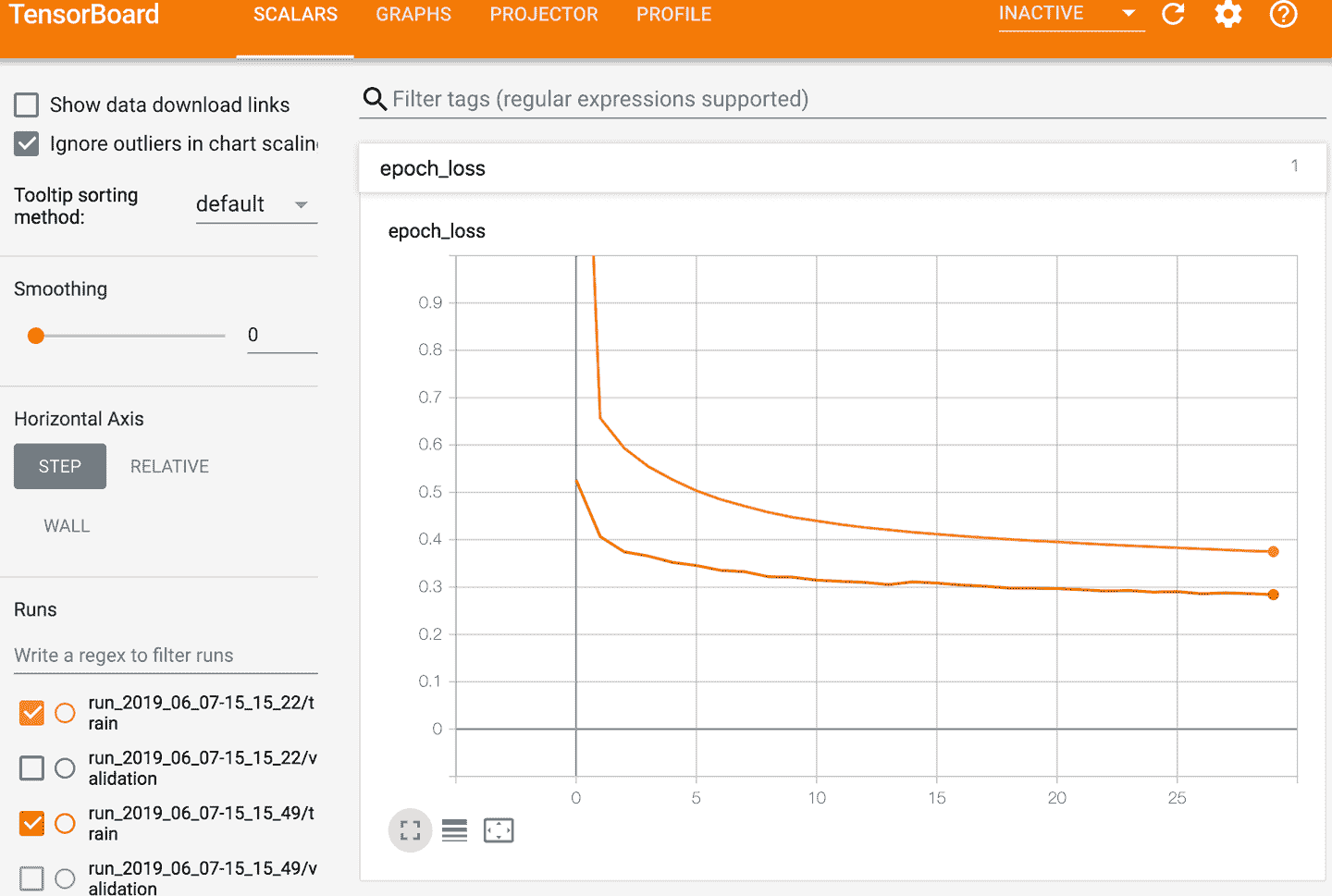test_logdir = get_run_logdir()
writer = tf.summary.create_file_writer(test_logdir)
with writer.as_default():
for step in range(1, 1000 + 1):
tf.summary.scalar("my_scalar", np.sin(step / 10), step=step)
data = (np.random.randn(100) + 2) * step / 100 # some random data
tf.summary.histogram("my_hist", data, buckets=50, step=step)
images = np.random.rand(2, 32, 32, 3) # random 32×32 RGB images
tf.summary.image("my_images", images * step / 1000, step=step)
texts = ["The step is " + str(step), "Its square is " + str(step**2)]
tf.summary.text("my_text", texts, step=step)
sine_wave = tf.math.sin(tf.range(12000) / 48000 * 2 * np.pi * step)
audio = tf.reshape(tf.cast(sine_wave, tf.float32), [1, -1, 1])
tf.summary.audio("my_audio", audio, sample_rate=48000, step=step)


### 微调神经网络的超参数

def build_model(n_hidden=1, n_neurons=30, learning_rate=3e-3, input_shape=):
model = keras.models.Sequential()
for layer in range(n_hidden):
optimizer = keras.optimizers.SGD(lr=learning_rate)
model.compile(loss="mse", optimizer=optimizer)
return model


keras_reg = keras.wrappers.scikit_learn.KerasRegressor(build_model)


KerasRegressor是通过build_model()将 Keras 模型包装起来的。因为在创建时没有指定任何超参数，使用的是build_model()的默认参数。现在就可以像常规的 Scikit-Learn 回归器一样来使用它了：使用fit()方法训练，使用score()方法评估，使用predict()方法预测，见下面代码：

keras_reg.fit(X_train, y_train, epochs=100,
validation_data=(X_valid, y_valid),
callbacks=[keras.callbacks.EarlyStopping(patience=10)])
mse_test = keras_reg.score(X_test, y_test)
y_pred = keras_reg.predict(X_new)


from scipy.stats import reciprocal
from sklearn.model_selection import RandomizedSearchCV

param_distribs = {
"n_hidden": [0, 1, 2, 3],
"n_neurons": np.arange(1, 100),
"learning_rate": reciprocal(3e-4, 3e-2),
}

rnd_search_cv = RandomizedSearchCV(keras_reg, param_distribs, n_iter=10, cv=3)
rnd_search_cv.fit(X_train, y_train, epochs=100,
validation_data=(X_valid, y_valid),
callbacks=[keras.callbacks.EarlyStopping(patience=10)])


>>> rnd_search_cv.best_params_
{'learning_rate': 0.0033625641252688094, 'n_hidden': 2, 'n_neurons': 42}
>>> rnd_search_cv.best_score_
-0.3189529188278931
>>> model = rnd_search_cv.best_estimator_.model


Hyperopt 一个可以优化各种复杂搜索空间（包括真实值，比如学习率和离散值，比如层数）的库。

HyperaskoptTalos 用来优化 Keras 模型超参数的库（前两个是基于 Hyperopt 的）。

Keras Tuner Google 开发的简单易用的 Keras 超参数优化库，还有可视化和分析功能。

Scikit-Optimize (skopt) 一个通用的优化库。类BayesSearchCV使用类似于GridSearchCV的接口做贝叶斯优化。

Spearmint 一个贝叶斯优化库。

Hyperband 一个快速超参数调节库，基于 Lisha Li 的论文《Hyperband: A Novel Bandit-Based Approach to Hyperparameter Optimization》

Sklearn-Deap 一个基于进化算法的超参数优化库，接口类似GridSearchCV

# 练习

1. TensorFlow Playground 是 TensorFlow 团队推出的一个便利的神经网络模拟器。只需点击几下，就能训练出二元分类器，通过调整架构和超参数，可以从直观上理解神经网络是如何工作的，以及超参数的作用。如下所示：

a. 神经网络学到的模式。点击左上的运行按钮，训练默认的神经网络。注意是如何找到分类任务的最优解的。第一个隐藏层学到了简单模式，第二个隐藏层将简单模式结合为更复杂的模式。通常，层数越多，得到的模式越复杂。

b. 激活函数。用 ReLU 激活函数代替 tanh，再训练一次网络。注意，找到解变得更快了，且是线性的，这归功于 ReLU 函数的形状。

c. 局部最小值的风险。将网络只设定为只有一个隐藏层，且只有 3 个神经元。进行多次训练（重置网络权重，点击Reset按钮）。可以看到训练时间变化很大，甚至有时卡在了局部最小值。

d. 神经网络太小的状况。去除一个神经元，只剩下两个。可以看到，即使尝试多次，神经网络现也不能找到最优解。模型的参数太少，对训练集数据欠拟合。

e. 神经网络足够大的状况。将神经元数设为 8，再多次训练神经网络。可以看到过程很快且不会卡住。这是一个重要的发现：大神经网络几乎从不会卡在局部最小值，即使卡住了，局部最小值通常也是全局最小值。但是仍然可能在平台期卡住相当长时间。

g. 再尝试尝试其它参数。

1. 用原始神经元（像图 10-3 中的神经元）画 ANN，可以计算A ⊕ B⊕表示 XOR 操作）。提示：A ⊕ B = (A ∧ ¬B ∨ (¬A ∧ B)

2. 为什么逻辑回归比经典感知机（即使用感知机训练算法训练的单层的阈值逻辑单元）更好？如何调节感知机，使其等同于逻辑回归分类器？

3. 为什么逻辑激活函数对训练 MLP 的前几层很重要？

4. 说出三种流行的激活函数，并画出来。

5. 假设一个 MLP 的输入层有 10 个神经元，接下来是有 50 个人工神经元的的隐藏层，最后是一个有 3 个人工神经元的输出层。所有的神经元使用 ReLU 激活函数。回答以下问题：

6. 输入矩阵X的形状是什么？

7. 隐藏层的权重向量W[h]和偏置项b[h]的形状是什么?

8. 输出层的权重向量W[o]和偏置项b[o]的形状是什么?

9. 输出矩阵Y的形状是什么？

10. 写出用X, W[h], b[h], W[o], b[o]计算矩阵Y的等式。

11. 如果要将邮件分为垃圾邮件和正常邮件，输出层需要几个神经元？输出层应该使用什么激活函数？如果任务换成 MNIST，输出层需要多少神经元，激活函数是什么？再换成第 2 章中的房价预测，输出层又该怎么变？

12. 反向传播是什么及其原理？反向传播和逆向 autodiff 有什么不同？

13. 列出所有简单 MLP 中需要调节的超参数？如果 MLP 过拟合训练数据，如何调节超参数？

14. 在 MNIST 数据及上训练一个深度 MLP。